Author: Oscar Cronquist Article last updated on October 08, 2019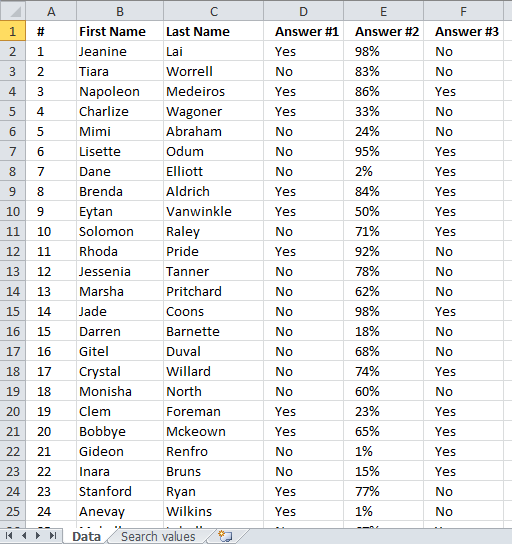This article explains how to filter a data set based on extremely many conditions in an Excel defined Table, in fact, this example has 1500 conditions. So many that it is tedious and time-consuming to enter or press with left mouse button on checkboxes in a Filter / Excel Table.

I have all conditions entered in a worksheet and I am going to utilize a formula to apply all conditions at once. This article was created to answer the following question:

I have problem, and o don't know how to solve it, I have data of almost 10000 forms, from which I have to find 1500, so it is very difficult to dig out 1500 one by one through Ctrl+F, is there any way to to put all the forms number at once and then find all those just by press with left mouse button oning. the primary key is Form Number.The "Data" worksheet contains the form numbers in column A, the remaining columns contain dummy data.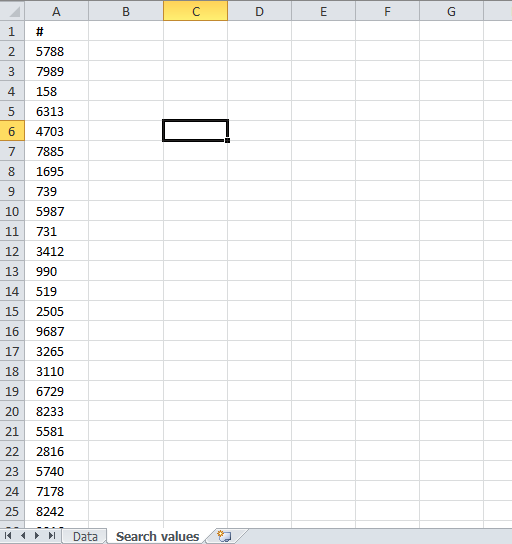The next sheet "Search values" contains the 1500 form numbers you want to use as conditions.

### Convert data set to an Excel defined Table

I will convert the data, in this example, to an Excel defined Table. You can also use an Excel Filter if you prefer that.

1. Select any cell in your data set.
2. Press Ctrl + T which is a shortcut for converting data to an Excel defined Table.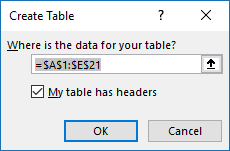3. Press OK button.

Excel now applies formatting to your data set indicating it is now an Excel defined Table.

### Search form numbers

It is now time to enter the formula, it will be automatically copied to cells below by the Excel defined Table. This is great saving us a little bit of time.

Formula in cell G2:

=COUNTIF('Search values'!\$A\$2:\$A\$1501, [@['#]])

Make sure you use absolute cell references. The COUNTIF function counts the number of values in cell range 'Search values'!\$A\$2:\$A\$1501 that is equal to the value in column #. Here is the function syntax:

COUNTIF(rangecriteria)

It returns 1 if the value in column # is found in the cell range containing conditions and 0 (zero) if not found. If there is more than one instance of a value in 'Search values'!\$A\$2:\$A\$1501 the formula may return a value greater than 1.

### Filter table

The Excel defined Table lets you select which values you want to filter, there is now only 1 or 0 (zero) to choose from which is much easier to base a filter on than 1500 different conditions.

1. Press with left mouse button on the black arrow at the top in column G which is the same column you entered the formula in.
2. Make sure only 1 is selected since this indicates that the value on the same row in column # is equal to one of the 1500 conditions specified in cell range 'Search values'!\$A\$2:\$A\$1501.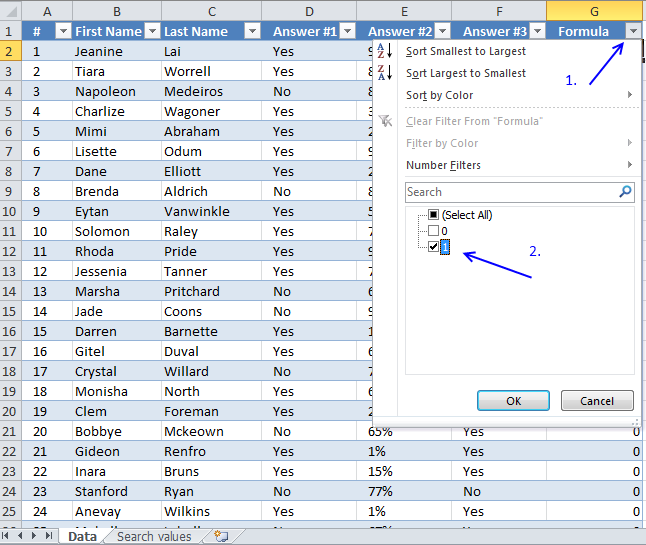3. Press OK button to apply the filter.

Below is a picture of the filtered Excel defined Table containing the 1500 form numbers,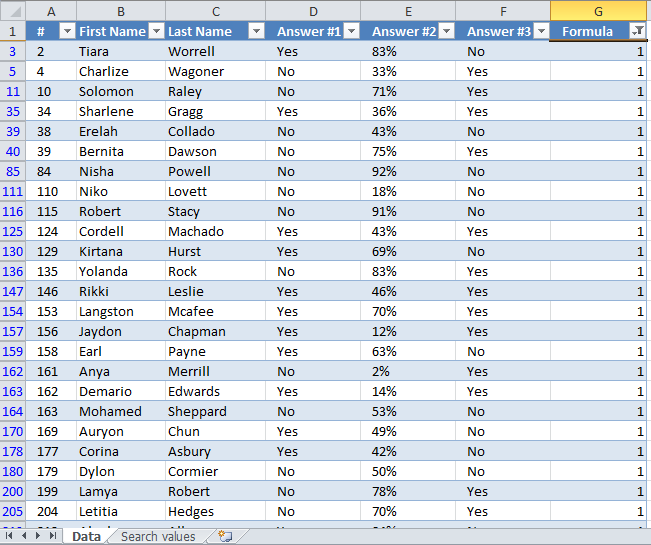### Get the Excel fileQuickly-search-a-table-using-many-criteria.xlsx

### Recommended articles

Vlookup a cell range and return multiple values

VLOOKUP a multi-column range and return multiple values.

Extract all rows that contain a value between this and that

Question: I have a list and I want to filter out all rows that have a value (Column C) that […]

Match two criteria and return multiple records

This article demonstrates how to extract records/rows based on two conditions applied to two different columns, you can easily extend […]

Extract duplicate records

This article describes how to filter duplicate rows with the use of a formula.  It is, in fact, an array […]

Filter unique distinct records

Table of contents Filter unique distinct row records Filter unique distinct row records but not blanks Filter unique distinct row […]